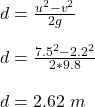## a volleyball is hit upward with an initial velocity of 7.5 m/s. calculate the displacement of the volleyball when its final velocity is 2.2m

Question

a volleyball is hit upward with an initial velocity of 7.5 m/s. calculate the displacement of the volleyball when its final velocity is 2.2m/s upwad

in progress 0
6 months 2021-07-22T02:50:01+00:00 1 Answers 5 views 0

The displacement of the volleyball is 2.62 m

Explanation:

Given;

initial velocity of the volleyball, u = 7.5 m/s

final velocity of the volleyball, v = 2.2 m/s

displacement of the volleyball, d = ?

Apply the following kinematic equation;

v² = u² – 2gd

2gd = u² – v²Therefore, the displacement of the volleyball is 2.62 m